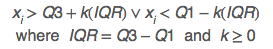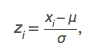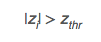{{announcement.body}}
{{announcement.title}}

# Four Techniques for Outlier Detection

DZone 's Guide to

# Four Techniques for Outlier Detection

### Learn from this article the four techniques to detect and optionally remove outliers from a dataset, including numeric outlier.

· AI Zone ·
Free Resource

Comment (0)

Save
{{ articles.views | formatCount}} Views

Ever been skewed by the presence of outliers in your set of data? Anomalies, or outliers, can be a serious issue when training Machine Learning algorithms or applying statistical techniques. They are often the result of errors in measurements or exceptional system conditions and therefore do not describe the common functioning of the underlying system. Indeed, the best practice is to implement an outlier removal phase before proceeding with further analysis.

But hold on there! In some cases, outliers can give us information about localized anomalies in the whole system; so the detection of outliers is a valuable process because of the additional information they can provide about your dataset.

There are many techniques to detect and optionally remove outliers from a dataset. In this blog post, we show an implementation in KNIME Analytics Platform of four of the most frequently used — traditional and novel — techniques for outlier detection.

## The Dataset and the Outlier Detection Problem

The dataset we used to test and compare the proposed outlier detection techniques is the well-known airline dataset. The dataset includes information about all US domestic flights between 2007 and 2012, such as departure time, arrival time, origin airport, destination airport, time on air, delay at departure, delay on arrival, flight number, vessel number, carrier, and more. Some of those columns could contain anomalies, i.e. outliers.

From the original dataset, we extracted a random sample of 1500 flights departing from Chicago O’Hare airport (ORD) in 2007 and 2008.

In order to show how the selected outlier detection techniques work, we focused on finding outliers in terms of average arrival delays at airports. Average arrival delay is calculated on all flights landing at a given airport. We are looking for those airports that show unusual average arrival delay times.

Figure 1 shows the outlier airports (detected by the isolation forest method). The blue circles represent airports with no outlier behavior while the red squares represent outlier airports. The average arrival delay time defines the size of the markers.

Notice that outlier airports are detected in both directions of the arrival delay scale: airports with frequent long arrival delays as well as airports with frequent small or even negative arrival delays. An example of such an airport with a negative average arrival delay (-17 min) is New Orleans Louis Armstrong International Airport (MSY).

Figure 1: Outlier airports as detected by the isolation forest technique. Notice the Spokane International Airport is the outlier airport with the highest average arrival delay. Also, notice that El Paso International Airport is the outlier airport with the lowest (negative!) average arrival delay.

The outlier status of the airports could then lead to a closer inspection of their recording processes and infrastructure.

Normalized average arrival delay times present the distribution shown in Figure 2, where normal distribution is followed very closely. The outlier airports cause the external ripples of this distribution.

Figure 2: Normalized average arrival delay vs. standard normal distribution

Topic. Detect outliers to prepare the dataset for Machine Learning training or to reveal interesting localized anomalies.

Data. Flights departing from Chicago O’Hare airport in the years 2007 and 2008 extracted from the airline dataset.

Methods. Four different outlier detection techniques: Numeric Outlier, Z-Score, DBSCAN and Isolation Forest.

## Four Outlier Detection Techniques

### Numeric Outlier

This is the simplest, nonparametric outlier detection method in a one-dimensional feature space. Here outliers are calculated by means of the IQR (InterQuartile Range).

The first and the third quartile (Q1, Q3) are calculated. An outlier is then a data point xi that lies outside the interquartile range. That is:Using the interquartile multiplier value k=1.5, the range limits are the typical upper and lower whiskers of a box plot.

This technique can easily be implemented in KNIME Analytics Platform using the Numeric Outliers node (Figure 3) which is one of the new features in KNIME Analytics Platform 3.6.

### Z-Score

Z-score is a parametric outlier detection method in a one or low dimensional feature space.

This technique assumes a Gaussian distribution of the data. The outliers are the data points that are in the tails of the distribution and therefore far from the mean. How far depends on a set threshold zthr for the normalized data points zicalculated with the formula:where where xi is a data point, μ is the mean of all xi and σ is the standard deviation of all xi.

An outlier is then a normalized data point which has an absolute value greater than zthr. That is:Commonly used zthr values are 2.5, 3.0 and 3.5.

### DBSCAN

This technique is based on the DBSCAN clustering method. DBSCAN is a nonparametric, density based outlier detection method in a one or multi-dimensional feature space.

In the DBSCAN clustering technique, all data points are defined either as Core Points, Border Points or Noise Points.

• Core Points are data points that have at least MinPts neighboring data points within a distance ε.
• Border Points are neighbors of a Core Point within the distance ε but with less than MinPts neighbors within the distance ε.
• All other data points are Noise Points, also identified as outliers.

Outlier detection thus depends on the required number of neighbors MinPts, the distance ε and the selected distance measure, like Euclidean or Manhattan.

### Isolation Forest

This is a nonparametric method for large datasets in a one or multi-dimensional feature space.

An important concept in this method is the isolation number.

The isolation number is the number of splits needed to isolate a data point. This number of splits is ascertained by following these steps:

• A point “a” to isolate is selected randomly.
• A random data point “b” is selected that is between the minimum and maximum value and different from “a”.
• If the value of “b” is lower than the value of “a”, the value of “b” becomes the new lower limit.
• If the value of “b” is greater than the value of “a”, the value of “b” becomes the new upper limit.
• This procedure is repeated as long as there are data points other than “a” between the upper and the lower limit.

It requires fewer splits to isolate an outlier than it does to isolate a nonoutlier, i.e. an outlier has a lower isolation number in comparison to a nonoutlier point. A data point is therefore defined as an outlier if its isolation number is lower than the threshold.

The threshold is defined based on the estimated percentage of outliers in the data, which is the starting point of this outlier detection algorithm.

This technique was implemented using the KNIME Python Integration and the isolation forest algorithm in the Python sklearn library. Below you can see the Python code used in the Python Script (1:1) node in Figure 3.

``````from sklearn.ensemble import IsolationForest
import pandas as pd
clf = IsolationForest(max_samples=100, random_state=42)
table = pd.concat([input_table['Mean(ArrDelay)']], axis=1)
clf.fit(table)
output_table = pd.DataFrame(clf.predict(table))``````

An explanation with images of this technique is available at https://quantdare.com/isolation-forest-algorithm/.

## Summary Table

This table summarizes the characteristics of the four outlier detection techniques described in this section:

Table 1: Summary table of the four outlier detection techniques described in the previous sections.

## Implementation in a KNIME Workflow

The KNIME workflow in Figure 3 implements the four proposed outlier detection techniques.

In the workflow, we:

2. Preprocess the data and calculate the average arrival delay per airport inside the Preproc metanode.
3. In the next metanode called Density of delay, we normalize the data and plot the density of the normalized average arrival delays against the density of a standard normal distribution.
4. Detect outliers using the four selected techniques.
5. Visualize the outlier airports in a map of the US in the MapViz metanode using the KNIME OSM integration.

Figure 3: KNIME Workflow implementing four outlier detection techniques: Numeric Outlier, Z-score, DBSCAN, Isolation Forest. This workflow is available on the KNIME EXAMPLES server under 02_ETL_Data_Manipulation/01_Filtering/07_Four_Techniques_Outlier_Detection/Four_Techniques_Outlier_Detection.

## The Detected Outliers

In Figures 4-7 you can see the outlier airports as detected by the different techniques.

The blue circles represent airports with no outlier behavior while the red squares represent airports with outlier behavior. The average arrival delay time defines the size of the markers.

A few airports are consistently identified as outliers by all techniques: Spokane International Airport (GEG), University of Illinois Willard Airport (CMI) and Columbia Metropolitan Airport (CAE). Spokane International Airport (GEG) is the biggest outlier with a very large (180 min) average arrival delay. This airport could therefore be a candidate for further inspection of the airport processes and infrastructure.

A few other airports however are identified by only some of the techniques. For example Louis Armstrong New Orleans International Airport (MSY) has been spotted by only the isolation forest and DBSCAN techniques.

Note that for this particular problem the z-score technique identifies the lowest number of outliers while the DBSCAN technique identifies the highest number of outlier airports.

Only the DBSCAN method (MinPts=3, ε=1.5, distance measure Euclidean) and the isolation forest technique (estimated percentage of outliers 10%) find outliers in the early arrival direction.

Numeric OutlierFigure 4: Outlier airports detected by numeric outlier technique

Z-ScoreFigure 5: Outlier airports detected by z-score technique

DBSCANFigure 6: Outlier airports detected by DBSCAN technique

Isolation ForestFigure 7: Outlier airports detected by isolation forest technique

## Summary

In this post, we have described and implemented four different outlier detection techniques in a one-dimensional space: the average arrival delay for all US airports between 2007 and 2008 as described in the airline dataset.

The four techniques we investigated are the numeric outlier, z-score, DBSCAN and isolation forest methods. Some of them work for one-dimensional feature spaces, some for low dimensional spaces, and some extend to high dimensional spaces. Some of the techniques require normalization and a Gaussian distribution of the inspected dimension. Some require a distance measure, and some the calculation of mean and standard deviation. The characteristics are all summarized in Table 1.

There are three airports that all the outlier detection techniques identify as outliers due to the large average arrival delay.

However, only some of the techniques (DBSCAN and isolation forest) could identify the outliers in the left tail of the distribution, i.e. those airports where, on average, flights arrived earlier than their scheduled arrival time.

## References

This workflow is available on the KNIME EXAMPLES server under 02_ETL_Data_Manipulation/01_Filtering/07_Four_Techniques_Outlier_Detection.

The theoretical basis for this blog post was taken from:

Santoyo, Sergio. (2017, September 12). A Brief Overview of Outlier Detection Techniques [Blog post].

https://towardsdatascience.com/a-brief-overview-of-outlier-detection-techniques-1e0b2c19e561

Topics:
machine learning algorithms ,anomaly detection ,machine learning ,data science

Comment (0)

Save
{{ articles.views | formatCount}} Views

Published at DZone with permission of Maarit Widmann , DZone MVB. See the original article here.

Opinions expressed by DZone contributors are their own.